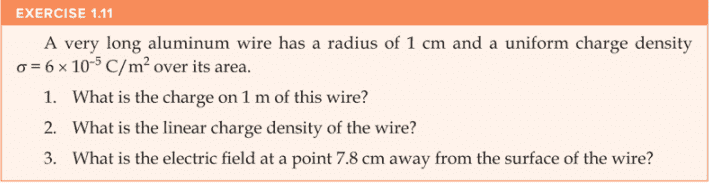# Gauss Law general physics 2 question

• DavidGarcia

#### DavidGarcia

Homework Statement
A very long aluminum wire has a radius of 1 cm and an uniform charge density of 6 x 10^-5 C/m^2 over its area. 1.) what is the charge on 1 m of this wire? 2.) What is the linear charge density of the wire? 3.) What is the electric field at a point 7.8 cm away from the surface of the wire?
Relevant Equations
E (electric field of sheet of charge) = uniform charge density / 2 (8.85 x 10^-12 C^2 / N*m^2 )
Electric flux = E(electric field) * A(area)
Q(Charge inside) = charge density * A(area)
E (electric field of a cylinder) = Charge per unit of length / (2*pi*r*(8.85 x 10^-12 C^2 / N*m^2))